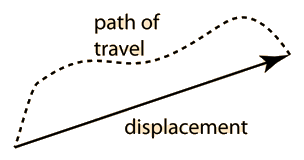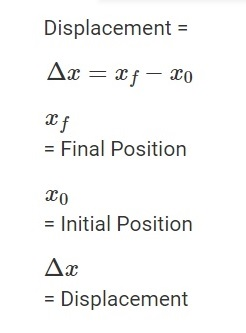# Position And Displacement Study Guide

INTRODUCTION

”Where are you?” is a question we’ve all been asked because of our poor navigational skills. In how many different ways can this question be answered? ‘I’m in front of the red truck,’ might be an answer. One might even mention your coordinates on the planet if you want to be more specific. There are numerous methods for determining your location. Normally, position refers to the location or placement of someone or something, but let’s look at the scientific definition of position.

## DEFINITION OF POSITION

• Position (x) can be defined as the location of an object at any given time.
• We should be able to define the position x of any item in order to explain its motion.
• To put it another way, we ought to identify its location in relation to the standard frame of reference.
• The random set of dimensions wherein the position and motions of an item are represented is referred to as a frame of reference.
• When describing the position of an object in relation to stationary objects on Earth, we frequently use Earth as the point of reference.
• An example for the position of an object may be where a soccer ball is in relation to the nearby player.Source

## DISPLACEMENT

• The definition of displacement is the change in the position of an object.
• The position of an object changes when it shifts relative to a reference frame.
• Displacement is the term for this shift in position.
• Examples of displacement can be – when a soccer ball moves to the right relative to a player or when a person moves toward the back of a car.Source

## DISPLACEMENT FORMULA

The shortest distance between the starting and final points is used to determine displacement, which favors straight-line paths over curved paths.Source

# SUMMARY

• Position (x) can be defined as the location of an object at any given time.
• The random set of dimensions wherein the position and motions of an item are represented is referred to as a frame of reference.
• Displacement is the change in the position of an object.

## FAQs

1. What is the relationship between position and displacement? Position (x) can be defined as the location of an object at any given time, and displacement is the difference between two position vectors.

2. How do you find the displacement of a position function?

The change in position of an object is called displacement. If the object moves from the position a(t1) to position a(t2) , then the displacement is a(t2) – a(t1) over the interval (ti , t2).

3. What are the similarities between position and displacement?

Position (x) can be defined as the location of an object at any given time, and Displacement is the change in position of an object. Both of these are vector quantities and have to be measured from the frame of reference.

We hope you enjoyed studying this lesson and learned something cool about Displacement And Position! Join our Discord community to get any questions you may have answered and to engage with other students just like you! We promise, it makes studying much more fun!😎

## REFERENCES

1. Position Formula: https://www.toppr.com/guides/physics-formulas/position-formula/. Accessed 8th April 2022.
2. Position and Displacement: https://www.ck12.org/physics/position-and-displacement/lesson/position-and-displacement-ppc/#:~:text=Position%20is%20the%20location%20of,from%20one%20time%20to%20another. Accessed 8th April 2022.
]]>